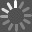The Theoretical Minimum What You Need to Know to Start Doing Physics Book - 2013

This book about "Classical Physics" and "classical mechanics" is an admirable attempt to give the layperson an introduction to physics which is "more than the Scientific American experience" and which is an alternative superior to the "childish anecdotes" ('t Hooft) imparted with physics textbooks written for high school students and for, say, premed college students. Still, it has some wrinkles which need to be ironed out with a new edition. For example, on p.77 it's claimed that "One condition for a local minimum is that the derivative of the function...is zero." But this claim about "a necessary condition" confounds the derivative of a function with the value given by that derivative---which is another function---when evaluated for some value of the domain. The function could be F(y)=y^3, in which case d/dy F(y)=3y^2. But 3y^2 does not necessarily take on a value of zero. The authors add to the confusion by claiming also that "d/dy F(y)=0" is "a sufficient condition" which "defines any stationary point". If so, then F(y)=c and there is no local minimum. Further, the 2nd derivative of F(y)=c is ALWAYS zero when the domain is the real numbers; it's never less than or greater than zero, as they imply it could be on p. 78. Again, the authors confounded the derivative with the concept of evaluating that derivative for some value of its domain. This is a strange mistake given that on p.113 they use the vertical bar to indicate the evaluation of a partial derivative at a specific value of the domain.

A more significant problem is the book's rush into the dungeon of determinism. It is, of course, important to recognize the importance of determinism in classical physics, but it doesn't follow that the teaching of physics should be restrained by the ball and chain of that philosophical error. In fact, the authors could have added to Lecture 1 an exercise in which the reader must write about and depict a dynamical law for the flipping of an ordinary coin in OUR world. Such an exercise would have directed the reader's attention toward the fact that the dynamical law of our world is not deterministic, at least in the sense supposed in classical mechanics, and the task of writing the details, subject to the limits of the reader's knowledge as it is in Lecture 1, would have provided a useful segue for later use when probability calculation becomes important to the physicist, and to the natural philosopher. The exercise would have called attention also to another important aspect of physics: It's preceeded by casual observation about regularities which everyone should notice and by attempts through philosophizing to arrive at a rigorous understanding of the dynamical law, or regularities, of our world.

[]
[]